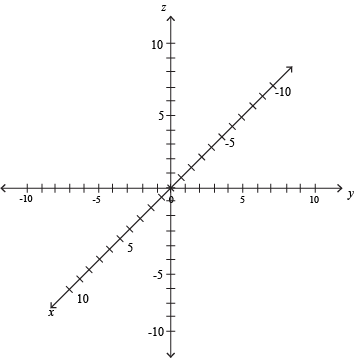This week in math class, we learned about a new type of coordinate planes and how to graph shapes on them. This is a 3D coordinate plane. It has three axises; x, y, and z. Since there are three axises, the ordered pair changes to an ordered triple. An example of an ordered triple would be (1, -5, 4). To find the distance and midpoint of a line on this type of graph is easier than you would think it would be. For the distance formula, all you do is add an extra part that includes the third axis. For the midpoint, it is the same thing, you do the same thing for the third axis as you do for the other two original axises.I hoped you learned something.